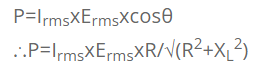# Choke Coil

A DC can be reduced by using a resistor, but while doing so considerable amount of electrical energy is lost in the form of heat. Similarly, AC can be controlled by using a suitable inductor or resistor. Once again using a resistor leads us to the loss of electrical energy. So instead of using a resistor we prefer a choke coil.
So the inductor used to control the ac without much loss of energy is called the choke coil.
However practical choke coil (inductor) is always accompanied by some resistance. This resistance can be considered to be in series with the inductor. So the power consumes is given by: